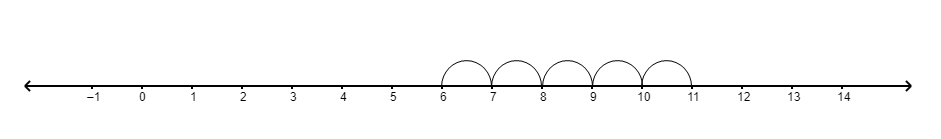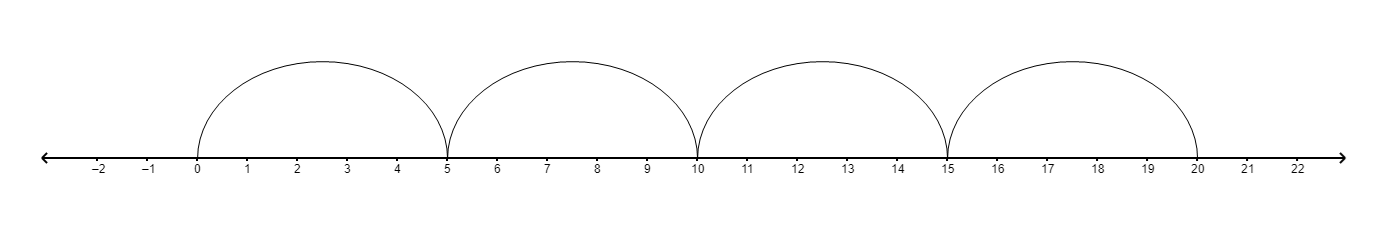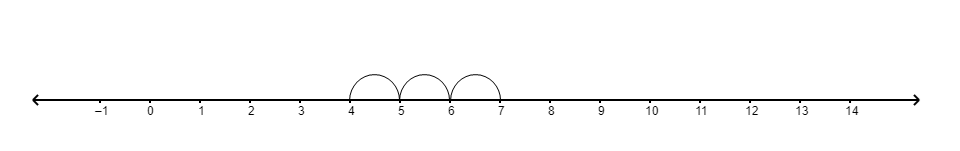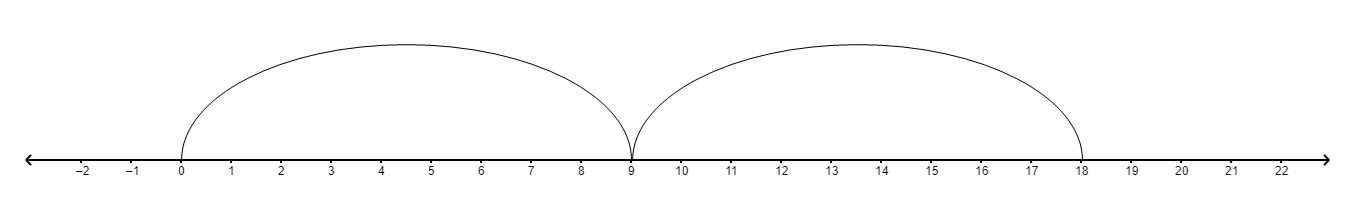# Represent number line for the following:$a) 6+5$b) $4 \times 5$c) $7-3$d) $2 \times 9$

Given:

$6 + 5$, $4 \times 5$, $7 - 3$ and $2 \times 9$.

To find:

We have to represent given values on the number line.

Solution:

(a) $6 + 5$

Step 1: First mark 6.

Step 2: Move 5 steps to the right.(b) 4 $\times$ 5

Starting from 0 we have to make 4 jumps and each jump is of 5 steps.(c) $7 - 3$

Step 1: First mark 7.

Step 2: Move 3 steps to the left.(d) 2 $\times$ 9

Starting from 0 we have to make 2 jumps and each jump is of 9 steps.Updated on: 10-Oct-2022

18 Views